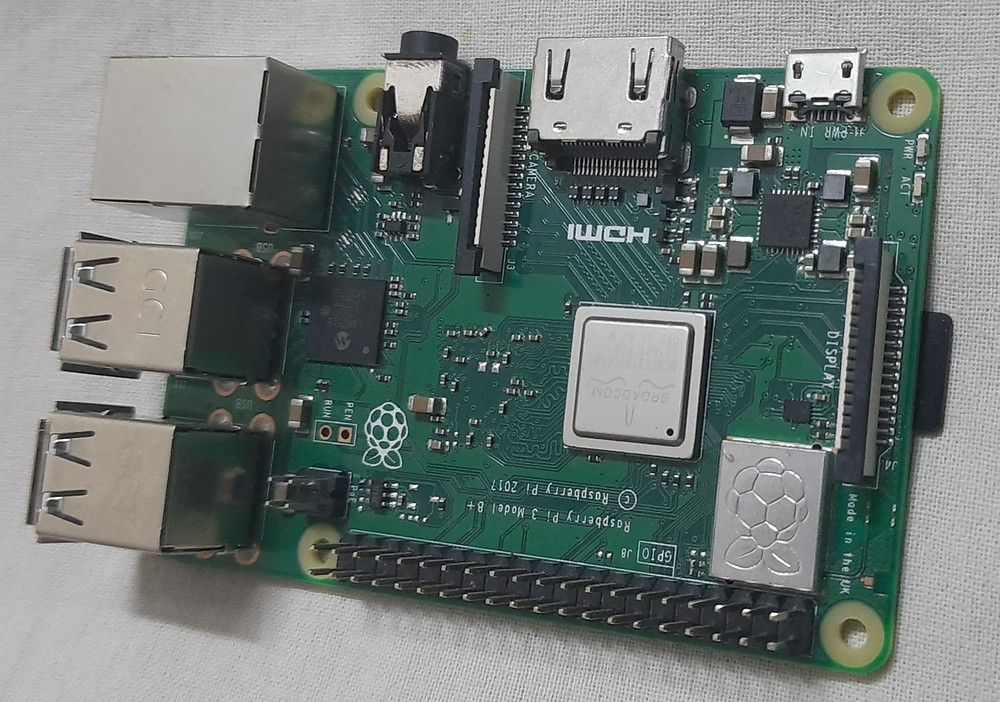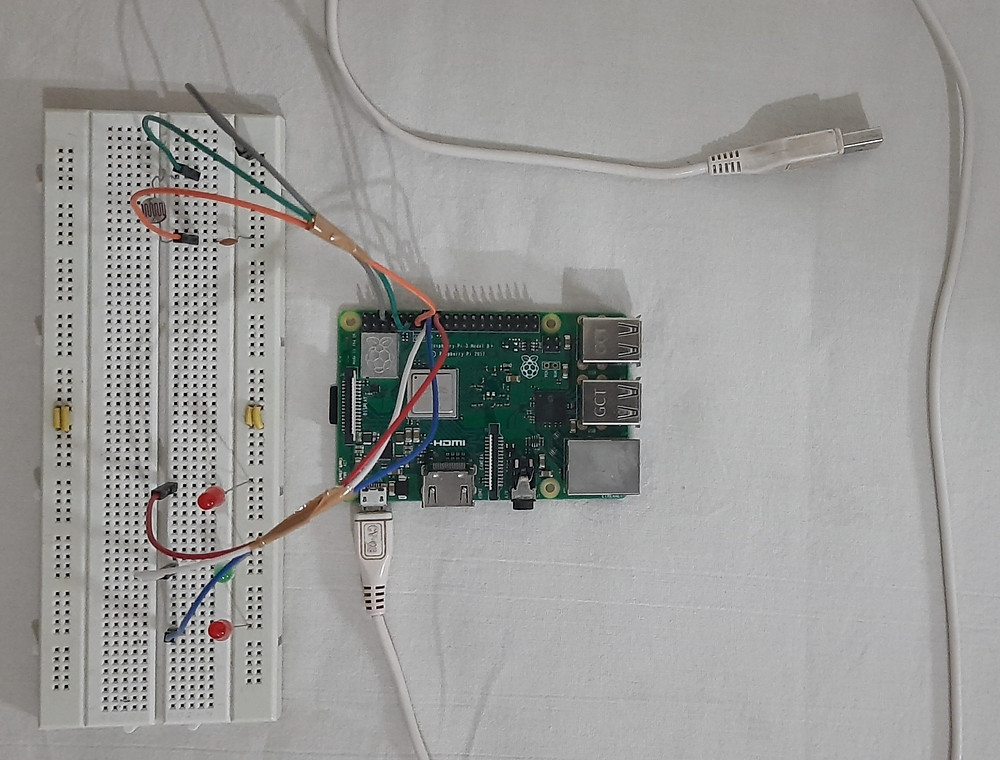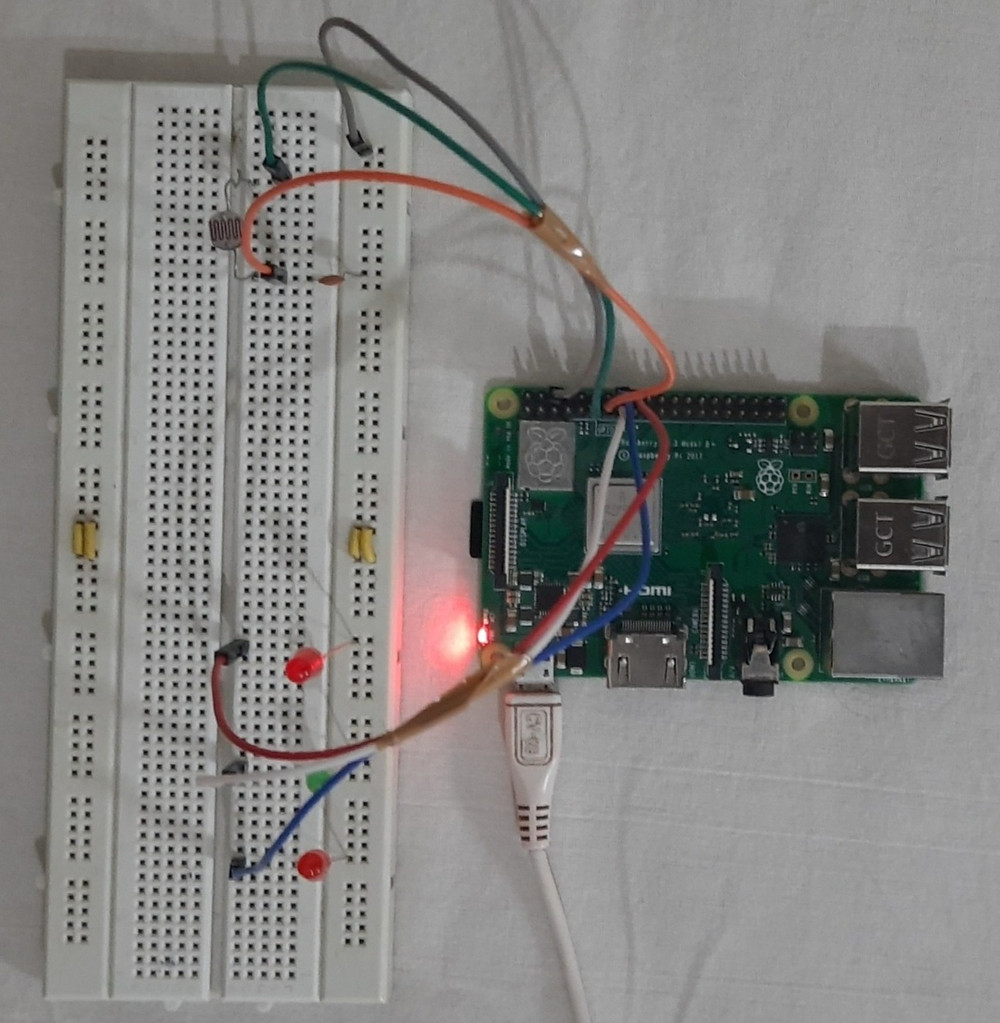# Smart Lighting System - IoT Prototype using Blynk App

Updated: Dec 15, 2020

The most standard entry into the world of automation is with a smart lighting system. Are the "Internet of Things" and Home Automation just lingos? In this project check out how we can bring home automation onto our finger-tips in our daily routine to make life much easier!

Here we use the #Blynk app which is a mobile application designed to control electrical appliances. The article provides step by step instructions involved in this interfacing which is pretty simple, starting from the list of electronic components required.

## Apparatus Required:

The list provides all the hardware and software components required for the interfacing.

### Hardware components:

1.LDR Sensor:

This is a cadmium-sulfide cell works based on the principle of Photoconductivity. It is basically a resistor whose resistance value changes depending upon the intensity of light. When the light falls on the surface of the senor, material conductivity varies as a result of which resistance also varies.LDR Sensor

Buy the LDR Sensor module from here.

2. Raspberry Pi 3:

We are using #Raspberrypi to connect with the LEDs, the #LDR sensor, and display the output. It contains several I/O pins using which we can connect the module and then by coding the Raspberrypi, we will receive the commands/signals that are sent from the mobile. This powerful credit-card-sized single-board computer can be used for many applications and supersedes the original Raspberry Pi Model.Raspberry Pi 3 Model B

Buy Raspberry Pi 3B+ from here.

3. Jumper wires:

These are simple connecting electrical wires, that are used to connect the ends without any soldering. The male wires have ends that can be connected to other ends and the female wires are the ones that have the receiving end. Grouping these wires a cable can be formed.Jumper Wires

4. USB Cable:

This USB cable is used to connect the Raspberry Pi to the power supply. It also helps in the transmission of data.USB Cable

5. Capacitor:

The resistance measured by the LDR sensor is done with the help of the time constant concept. The time required to charge a capacitor is equal to the multiplied value of resistance of the circuit and capacitance. Here, a 0.1 uF capacitor is used.Ceramic Capacitor (0.1 micro)

The breadboard is the basic component of any circuit building process. All components, be it input sensors or output display devices are connected to the #Arduino or any other microcontroller using wired connections through a breadboard. The holes in the breadboard are in series. There are various sizes like full-sized, half-sized, and mini breadboards.### Software components:

7.SSH Terminal:

The SHH Terminal is used in this project to connect the Raspberry Pi module to the terminal of another computer or peripheral devices. Encrypted data transfer between two computers is supported by this terminal.SSH Terminal

8.Python IDE:

Programming is done in #Python language in an IDE (Integrated Development Environment) which is a platform where we can not only write the code but also run, and test the working.Python IDLE IDE 3.7

### CIRCUIT DIAGRAM:Hardware Circuit Diagram

In this section, let's see all the connections of the circuit with all the components whose brief introduction is given above.

What goes where?

• The Blue line connects one terminal of the #LDRsensor to GPIO 17 pin, of the Raspberry Pi module, that is the board pin number 11. The other end of the sensor is connected to one end of the capacitor.

• The orange line connects this end to the GPIO 27 pin of the Raspberry Pi 3, which is the board pin number 13.

• Another end of the capacitor is connected by the Black wire to the ground (GND) pin of the Pi module.

NOTE: Basically these pin numberings are useful for writing python scripts for the Raspberry Pi.

Now the LEDs which are an alternative for the lights and bulbs at our home, here we see that the GPIO pins, the board pin number 18, 15, and 16 of the Raspberry Pi are connected to the LEDs here in the circuit. The GPOIs connected with the resistors, the resistors in turn connected with the anode of the LED, and the cathode connected to the ground(GND).Hardware Circuit Unpowered

The above image depicts the hardware circuit when unpowered, before any code implementation.Hardware Circuit Powered-Up

Here, the above image depicts the hardware circuit when it is powered up, the setup is ready for the program to run using the raspberry Pi terminal.

### Code Explanation:

For GPIO pins set up and output declaration RPi. GPIO library is imported. The next command imports the LDR values from the ldr_pr function file. The Blynk Libraries are imported, one can download them through the internet and use them in their code. To read the values of the virtual pins on the app to the Raspberry Pi board, the Blynkapi is imported. To use some system-specific parameters sys library is imported.

```import RPi.GPIO as GPIO
from ldr_pr import LDR
import BlynkLib
from blynkapi import Blynk
import sys```

Then here we have the Auth key, where the authentication would be entered. This authentication key would be your email, whenever you create a project the email registered with the Blynk app would be receiving the email with the project title and the authentication code. One has to enter that code here.

`BLYNK_AUTH = 'QR3RkZ_bA7ieCMqtOTZrArhFR8IWV5ud'`

Then we move to initialize the API functions here. Here we are declaring variables LED_1, LED_2 and so on which will be holding the function value, that is the Blynk function from the Blynk API module, with the Blynk authentication key along with the virtual pin setup on the app. All_LED is for the LED status and DEC is for the decision making, for the lighting volt button which we have included in the Blynk app.

NOTE: We use virtual pins to show the data on the Blynk app.

```LED_1 = Blynk(BLYNK_AUTH, pin = "V5")
LED_2 = Blynk(BLYNK_AUTH, pin = "V7")
LED_3 = Blynk(BLYNK_AUTH, pin = "V6")
All_LED = Blynk(BLYNK_AUTH, pin = "V3")
DEC = Blynk(BLYNK_AUTH, pin = "V8")```

Based on the above values we will be making some of the computations and check out with the code.

Here we initialize the Blynk app or the Blynk commands.

`blynk = BlynkLib.Blynk(BLYNK_AUTH)`

For writing on the virtual pins, we have a function here my_write commands required to execute the smart mode are initiated. We set up the GPOI pins at GPIO.BOARD, we can do for GPIO.BCM as well. We then set the warnings as false. Then we initialize the GPIO pins connected with the LEDs and set them up as output pins 18,15 and 16 respectively.

```@blynk.VIRTUAL_WRITE(1)

def my_write():
GPIO.setmode(GPIO.BOARD)
GPIO.setwarnings(False)
GPIO.setup(18,GPIO.OUT)
GPIO.setup(15,GPIO.OUT)
GPIO.setup(16,GPIO.OUT)
```

Now here in the for loop we record the values of LDR, take five of the values and average it. Then we will be putting the average value back on to the Blynk app, now the virtual pin 2 here is used by the gauge which beholding the LDR values in the app.

``` p=0
for i in range(0,5):
p=p+LDR(11,13)
p_avg = p/5
blynk.virtual_write(2, '{:.2f}'.format(p_avg))
#print(p_avg)```

Once the above is done we make our decisions here, depending on the LDR values along with the threshold we compare them and set up the GPIO pins high or low accordingly. For if here the p_avg greater than 500 then switch on all the lights, we create a value=1 for LED status purpose and virtual write on pin 3 with the value assigned earlier.

```if p_avg > 500:
GPIO.output(18, GPIO.HIGH)
GPIO.output(15,GPIO.HIGH)
GPIO.output(16,GPIO.HIGH)
value=1
blynk.virtual_write(3, value)```

Here is the else statement, the LED status is set to zero. So this was about the my_write function.

```else:
GPIO.output(18, GPIO.LOW)
GPIO.output(15,GPIO.LOW)
GPIO.output(16,GPIO.LOW)
value=0
blynk.virtual_write(3, value)```

In this function, commands required to execute the manual mode are initiated. Similarly, GPIO pins are initiated as mentioned above. The get_val() function, assigns specific values for each LED in the Blynk app. For LED 1, if the value is 1 the GPIO pin is in a high state. If not, it should be a low state. Similar authentication is followed for the other 2 LEDs.

```@blynk.VIRTUAL_READ(4)
GPIO.setmode(GPIO.BOARD)
GPIO.setwarnings(False)
GPIO.setup(18,GPIO.OUT)
GPIO.setup(15,GPIO.OUT)
GPIO.setup(16,GPIO.OUT)
first_led=LED_1.get_val()
second_led=LED_2.get_val()
third_led=LED_3.get_val()
ld_1=int(float(first_led))
ld_2=int(float(second_led))
ld_3=int(float(third_led))
if ld_1==1:
GPIO.output(18, GPIO.HIGH)
else:
GPIO.output(18,GPIO.LOW)

if ld_2==1:
GPIO.output(15, GPIO.HIGH)
else:
GPIO.output(15,GPIO.LOW)

if ld_3==1:
GPIO.output(16, GPIO.HIGH)
else:
GPIO.output(16,GPIO.LOW)

```

Now we have the button status of the lighting mode. As we did in the LEDs section and the read function, we will be doing the same way to get the value of the switch, and then depending on the value, we run the specific program. blynk.run() would run all the commands initialized to the blynk functions. When the keyboard Interrupt is given we initialize the system-specific parameter and end the program.

```try:
while True:
dc=DEC.get_val()
dec=int(float(dc))
if dec==0:
my_write()

else:
blynk.run()

except KeyboardInterrupt:
sys.exit(0)```

### COMPLETE CODE:

```import RPi.GPIO as GPIO
from ldr_pr import LDR
import BlynkLib
from blynkapi import Blynk
import sys

BLYNK_AUTH = 'QR3RkZ_bA7ieCMqtOTZrArhFR8IWV5ud'

LED_1 = Blynk(BLYNK_AUTH, pin = "V5")
LED_2 = Blynk(BLYNK_AUTH, pin = "V7")
LED_3 = Blynk(BLYNK_AUTH, pin = "V6")
All_LED = Blynk(BLYNK_AUTH, pin = "V3")
DEC = Blynk(BLYNK_AUTH, pin = "V8")

blynk = BlynkLib.Blynk(BLYNK_AUTH)

@blynk.VIRTUAL_WRITE(1)

def my_write():
GPIO.setmode(GPIO.BOARD)
GPIO.setwarnings(False)
GPIO.setup(18,GPIO.OUT)
GPIO.setup(15,GPIO.OUT)
GPIO.setup(16,GPIO.OUT)
p=0
for i in range(0,5):
p=p+LDR(11,13)
p_avg = p/5
blynk.virtual_write(2, '{:.2f}'.format(p_avg))
#print(p_avg)
if p_avg > 500:
GPIO.output(18, GPIO.HIGH)
GPIO.output(15,GPIO.HIGH)
GPIO.output(16,GPIO.HIGH)
value=1
blynk.virtual_write(3, value)

else:
GPIO.output(18, GPIO.LOW)
GPIO.output(15,GPIO.LOW)
GPIO.output(16,GPIO.LOW)
value=0
blynk.virtual_write(3, value)

GPIO.setmode(GPIO.BOARD)
GPIO.setwarnings(False)
GPIO.setup(18,GPIO.OUT)
GPIO.setup(15,GPIO.OUT)
GPIO.setup(16,GPIO.OUT)
first_led=LED_1.get_val()
second_led=LED_2.get_val()
third_led=LED_3.get_val()
ld_1=int(float(first_led))
ld_2=int(float(second_led))
ld_3=int(float(third_led))
if ld_1==1:
GPIO.output(18, GPIO.HIGH)
else:
GPIO.output(18,GPIO.LOW)

if ld_2==1:
GPIO.output(15, GPIO.HIGH)
else:
GPIO.output(15,GPIO.LOW)

if ld_3==1:
GPIO.output(16, GPIO.HIGH)
else:
GPIO.output(16,GPIO.LOW)

try:
while True:
dc=DEC.get_val()
dec=int(float(dc))
if dec==0:
my_write()

else:
blynk.run()

except KeyboardInterrupt:
sys.exit(0)```

This is the code where the LDR values are taken from which are based on the time constant concept. We get the output of the resistance of the sensor, depending on the luminous intensity around us. Also in general we notice the luminous intensity is inversely proportional to the resistance values.

```import RPi.GPIO as GPIO
import time

def LDR(cpin,lpin):
GPIO.setmode(GPIO.BOARD)
cap=0.0000001
GPIO.setup(cpin, GPIO.OUT)
GPIO.setup(lpin, GPIO.OUT)
GPIO.output(cpin, False)
GPIO.output(lpin, False)
time.sleep(0.2)
GPIO.setup(cpin, GPIO.IN)
time.sleep(0.2)
GPIO.output(lpin, True)
starttime=time.time()
endtime=time.time()
while (GPIO.input(cpin) == GPIO.LOW):
endtime=time.time()
measureresistance=endtime-starttime
return(res/10)

```

ldr_blynk (Main)
.txt
TXT • 2KB

ldr_pr (Support Func)
.txt
TXT • 653B

Note: Please change the file format to .py(python file) before you upload it to Raspberry Pi.

### Working:

We have to configure our Blynk app with respective buttons to show the output. Create a new project and select the device Raspberry Pi 3. Enter the value display settings and allocate virtual buttons for each LED. The virtual buttons are used to display the data on the app.

• The V5 button is for LED 1.

• The V7 button is for LED 2.

• The V6 button is for LED 3.

• V8 button is to select either smart mode or the manual mode.

In Smart mode, the LEDs get ON once the resistance value is above the default threshold value. Here, the threshold value is 500 ohm. In manual mode, irrespective of the threshold value the ON/OFF of the LEDs is determined manually.

After the execution of the code, now let us check the working of the circuit. Now the setup is in manual mode.

MANUAL MODE:

When the virtual button for LED 1 is ON, the corresponding output is:Manual_LED_ON

When the virtual button for LED 2 is ON, the output is:Manual_LED_2_ON

Similarly, when the virtual button for LED 3 is ON, the output is:Manual_LED_3_ON

When all the three virtual buttons are ON, the output is:Manual_ALL_ON

When all the three virtual buttons are OFF, the output is:Manual_ALL_OFF

We can also perform operations such as all buttons are OFF, only 2 buttons in ON state, and obtain the respective output.

SMART MODE:

Now the setup is switched to the smart mode. At first, all three LEDs are glowing because the LDR value is above the threshold value (500 ohms).SMART_LED_ON

When the mobile flashlight is on, the LDR value starts decreasing. At some point, it gets below the default threshold value and all the LEDs get OFF.SMART_LED_OFF

For better understanding watch the video given below.

Video By - Sai Parimal

For most of us controlling lights was all about flipping lots of switches, but now we know how smart automated lighting revolutionizes the way we experience lighting. This article lay as the basement for the construction of greater ideas. So, you can use this wide range of automation ideas or creative ideas of your own. What are you waiting for? Let's get started.